Courses

# Adiabatic Process - Thermodynamic Chemistry Notes | EduRev

## Physical Chemistry

Created by: Asf Institute

## Chemistry : Adiabatic Process - Thermodynamic Chemistry Notes | EduRev

The document Adiabatic Process - Thermodynamic Chemistry Notes | EduRev is a part of the Chemistry Course Physical Chemistry.
All you need of Chemistry at this link: Chemistry

Adiabatic reversible work, heat and energy

We know that         PVr = constant = K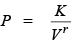we know,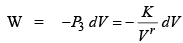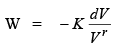Because process is reversible than integration will possible from V1 to V2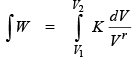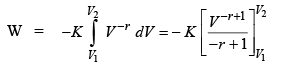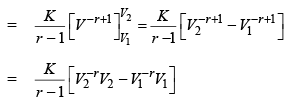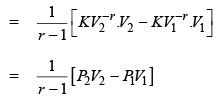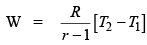PV= K
P1V1r = K
P2V2r = K
P1 = KV1–r
P2 = KV2–r
P1V1 = RT1
and P2V2 = RT2

Another way to find the work done in adiabatic process.

We know that d∵ = dU – ω
d∵ = 0 because process is adiabatic
dU = ω
⇒ ω = dV = CVdT
and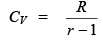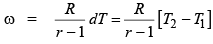d∵ = dU – w
d∵ = 0 for adiabat ic process

From first law
dq = dU – ω
∵ dq = 0
⇒ 0 = dU– ω

⇒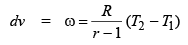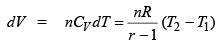and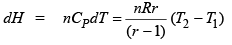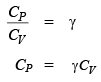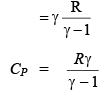Problem. A gas expands isothermally against a constant external pressure of 1 atm from a volume of 10 dm3 to a volume of 20 dm3. In this process it absorbs 800 J of thermal energy from its surrounding calculate DU for the process in joules (because process is irreversible)

Sol.
W = –P(V2 – V1)
= –1 atm (20 dm3 – 10 dm3) = –10 dm3 atm
∵ R = 8.314 JK–1 mol–1 = 8.02 × 10–2 dm3 atm K–1 mol–1
∴ 1 dm3 atm =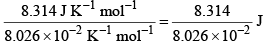1 dm3 atm = 1.013 × 102 J

Put this value in equation we get

W = – 10 dm3 atm
= – 10 × 1.013 × 10J = –1013 J
i.e. W = –1013 J

Problem.  (a)  2.0 mole of an ideal diatomic gas at 300 K and 0.507 MPa are expanded adiabatically to a final pressure of 0.203 MPa against a constant pressure of 0.101 MPa. Calculate the final temperature, q, w, ΔU & ΔH.
Sol.
(a) For an adiabat ic process q = 0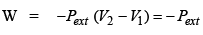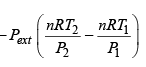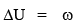i.e.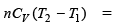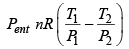CV for a diatomic molecule =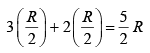(assuming no contribution for vibration)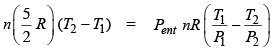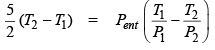Substituting the values, we get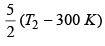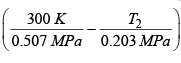Solving for T2, we get

T2 = 270 K

thus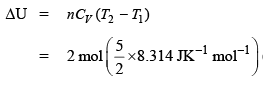(270 K -300 K)

= –1247.1 J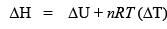= –1247.1 J + 2 mol × 8.314 JK–1 mol–1 (–30 K)
= –1745.9 J

or              CP – CV = R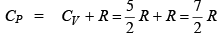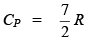and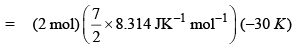= –1745.9 J

Note

Degree of freedom: There are three types of degree of freedom

(1) Translat ional (2) Rotational (3) Vibrational

⇒ If total no. of atoms are N then no. of degree of freedo m is 3 N.
⇒ For any atom or molecule the degree of freedom for translat ional is three (3).
⇒ For any atom or linear mo lecule the degree o f freedom for is 2 (two) and for non linear it is three (3).
⇒ For any atom or linear mo lecule the degree of freedom for vibrat ional is (3N – 5) and for non linear it is (3N – 6).
⇒ The energy contribution for each degree of freedom is 1/2 KT/molecule or 1/2 RT/mole.
⇒ The vibrational degree of freedo m is affected by temperature and it is 0.2 RT at room temperature and RT at high temperature.

Problem.  Calculate CV for Na, Cl2 & SO2?
Sol.
Total internal energy (U) for linear mo lecule is: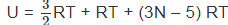(at high temperature)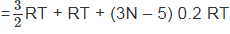(at room temperature)

Total energy (U) for non linear molecular is: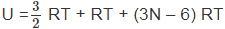(at high temperature)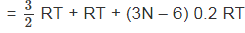(at room temperature)

We know that
⇒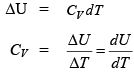CV for Na: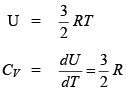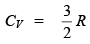CV for Cl2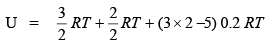(for linear molecule)

= 2.7 RT
CV = 2R

Cfor SO2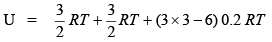for non linear molecule)
= 3.3 RT
CV = 3.3 R

Problem.  20 gm of N2 at 300 K is compressed reversible and adiabatically from 20 dm3 to 10 dm3. Calculate the final temperature, q, w, ΔH & ΔU.
Sol.
Amount of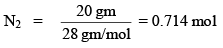i.e., n = 0.714 mol
T1 = 300 K
V= 20 dm3
V2 = 10 dm3
T= ?

T1(V1)r-1 = T2 (V2)r -1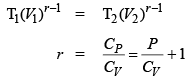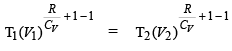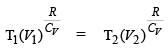Thus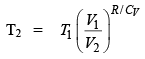and for linear molecule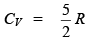Thus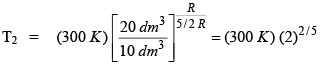T1 = 300 K × 1.32 = 396 K
Hence,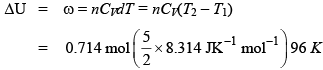= 1424.69 J
ΔH = nCP(T2 - T1)

= 0.714 mol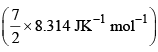96 K

= 1994.56 J

Offer running on EduRev: Apply code STAYHOME200 to get INR 200 off on our premium plan EduRev Infinity!

,

,

,

,

,

,

,

,

,

,

,

,

,

,

,

,

,

,

,

,

,

;